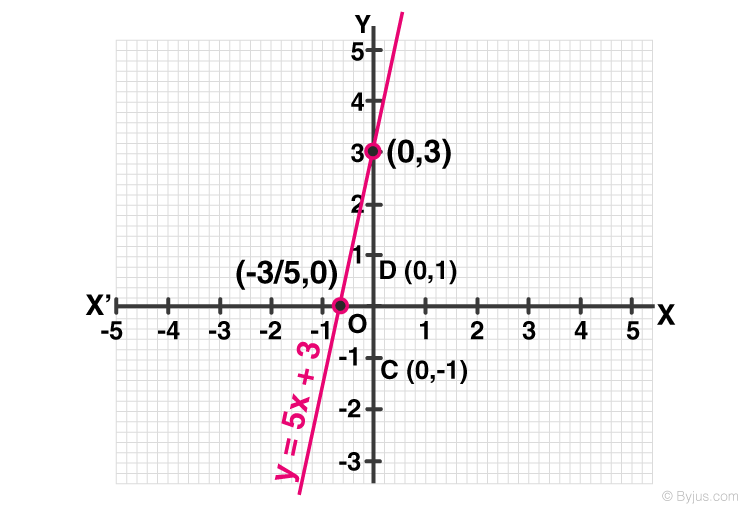Newbie

# The taxi fare in a city is as follows: For the first kilometer, the fare is ₹8 and for the subsequent distance it is ₹5 per km. Taking the distance covered as x km and total fare as ₹ y, write a linear equation for this information, and draw its graph.Q.4

• 0

Find the simplest solution of ncert class 9th solution of linear equations in two variables of exercise 4.3 question number 1(4). Sir please help me to solve this question in a easiest and best way. Please help me to solve this question in a simplest method. Q.4

Share

1. Solution:

Given,

Total distance covered = x

Total fare = y

Fare for the first kilometer = 8 per km

Fare after the first 1km = 5 per km

If x is the total distance, then the distance after one km = (x-1)km

i.e., Fare after the first km = 5(x-1)

According to the question,

The total fare = Fare of first km+ fare after the first km

y = 8+5(x-1)

y = 8+5(x-1)

y = 8+5x – 5

y = 5x+3

Solving the equation,

When x = 0,

y = 5x+3

y = 5×0+3

y = 3

When y = 0,

y = 5x+3

o = 5x+3

5x = -3

x = -3/5

 x y 0 3 -3/5 0

The points to be plotted are (0, 3) and (-3/5, 0)• 0